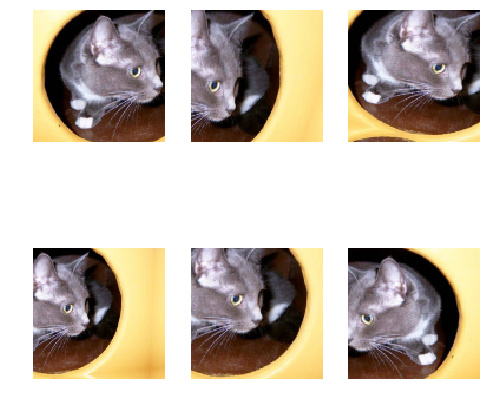# Augmentation in Part 1 Lesson 1 v2 using fastai v1.0

Hi

I am trying to use Kaggle GPU instead of paid services. Since lesson 1 is v2, and kaggle has fast.ai v1.0, I am kinda stuck heavily. Especialy in below code for past few hours.

``````tfms = tfms_from_model(resnet34, sz, aug_tfms=transforms_side_on, max_zoom=1.1)
def get_augs():
data = ImageClassifierData.from_names_and_array(
path=PATH,
fnames=fnames,
y=labels,
classes=['dogs', 'cats'],
test_name='test',
tfms=tfms,
num_workers=1,
bs=2
)
x,_ = next(iter(data.aug_dl))
return data.trn_ds.denorm(x)
ims = np.stack([get_augs() for i in range(6)])
plots(ims, rows=2)
``````

Not only that, I could not find random transformation methods to pass to ImageDataBunch without image argument like fastai 0.7, but also I am unable to get plot the augmented images because there is no aug_dl or denorm to operate upon or use. Below is my incomplete broken attempt.

``````tfms = get_transforms(max_zoom=1.1)
def get_augs():
data_a = ImageDataBunch.from_folder(
path,
ds_tfms=tfms,
size=sz,
num_workers=1)

x = next(iter(data_a.train_dl.x.items))
#     PIL.Image.open(x)
return x
ims = np.stack([get_augs() for i in range(6)])
plots(ims, rows=2)
``````

Can any one kindly help??

# Update 1:

This is what I have come up so far. Please help correcting or improving it if any issue.

``````import matplotlib.pyplot as plt
from random import randint

# fastai v0.7 definition for transforms_side_on not available in fastai v1.0
#transforms_basic    = [RandomRotate(10), RandomLighting(0.05, 0.05)]
#transforms_side_on  = transforms_basic + [RandomFlip()]

def get_augs():
rand_deg = randint(0,10)
rand_light = randint(0,5)/100
tfms = get_transforms(xtra_tfms = [rotate(degrees=rand_deg),flip_lr()], max_zoom=1.1, max_lighting=rand_light)
data_a = ImageDataBunch.from_folder(
path,
ds_tfms = tfms,
size=sz,
num_workers=1)

x,_ = next(iter(data_a.train_ds))
return x
ims = np.stack([get_augs() for i in range(6)])
%matplotlib inline
# plt.imshow(image2np(ims.data))
# plt.show()
fig, axr = plt.subplots(2,3, figsize=(8,8))
k = 0
for i in range(2): # rows
for j in range(3): # cols
ims[k].show(ax=axr[i,j])
k += 1
``````

output: